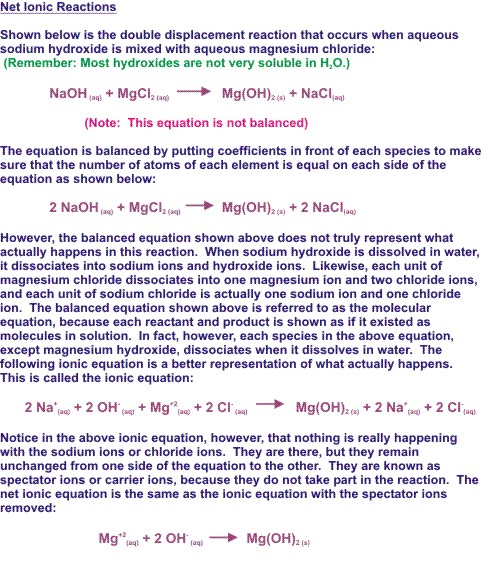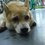# Balancing chemical equationsBalancing a chemical equation:

To balance the given or any chemical equation, follow these steps:

Fe(s) + H2O(l) ----> FE3O4 (s)+ H2 (g)

(1) Write the number of atoms of elements present in reactants and in products.

(2) Balance the atom which is the maximum in number; on either side of chemical equation.

In this equation, the number of oxygen atom is the maximum on Right Hand Side(RHS)

To balance the oxygen one needs to multiply the oxygen on the Left Hand Side (LHS)by 4; so that the number of oxygen atoms becomes equal on both sides.

Fe(s) + 4 x H2O(l) ----> FE3O4 (s)+ H2 (g)

(3) Now, the number of hydrogen atoms becomes 8 on the LHS; which is more than that on the RHS. To balance it, one needs to multiply the hydrogen on the RHS by 4.

Fe(s) + 4 x H2O(l) ----> FE3O4 (s)+ 4 x H2 (g)

(4) After that number of oxygen and hydrogen atoms becomes equal on both sides. The number of iron is one on the LHS, while it is three on the RHS. To balance it, multiply the iron on the LHS by 3.

3 x Fe(s) + 4 x H2O(l) ----> FE3O4 (s)+ 4 x H2 (g)

(5) Now the number of atoms of each element becomes equal on both sides. Thus, this equation becomes a balanced equation.

3Fe(s) + 4H2O(l) ----> FE3O4 (s)+ 4H2 (g)

                                Question


On heating blue coloured powder of copper (II) nitrate in a boiling tube, copper oxide (black), oxygen gas and a brown gas X is formed

(a) Write a balanced chemical equation of the reaction.

(b) Identify the brown gas X evolved.

(c) Identify the type of reaction.Note by Nicole Ling
7 years, 5 months ago

This discussion board is a place to discuss our Daily Challenges and the math and science related to those challenges. Explanations are more than just a solution — they should explain the steps and thinking strategies that you used to obtain the solution. Comments should further the discussion of math and science.

When posting on Brilliant:

• Use the emojis to react to an explanation, whether you're congratulating a job well done , or just really confused .
• Ask specific questions about the challenge or the steps in somebody's explanation. Well-posed questions can add a lot to the discussion, but posting "I don't understand!" doesn't help anyone.
• Try to contribute something new to the discussion, whether it is an extension, generalization or other idea related to the challenge.

MarkdownAppears as
*italics* or _italics_ italics
**bold** or __bold__ bold
- bulleted- list
• bulleted
• list
1. numbered2. list
1. numbered
2. list
Note: you must add a full line of space before and after lists for them to show up correctly
paragraph 1paragraph 2

paragraph 1

paragraph 2

[example link](https://brilliant.org)example link
> This is a quote
This is a quote
    # I indented these lines
# 4 spaces, and now they show
# up as a code block.

print "hello world"
# I indented these lines
# 4 spaces, and now they show
# up as a code block.

print "hello world"
MathAppears as
Remember to wrap math in $$ ... $$ or $ ... $ to ensure proper formatting.
2 \times 3 $2 \times 3$
2^{34} $2^{34}$
a_{i-1} $a_{i-1}$
\frac{2}{3} $\frac{2}{3}$
\sqrt{2} $\sqrt{2}$
\sum_{i=1}^3 $\sum_{i=1}^3$
\sin \theta $\sin \theta$
\boxed{123} $\boxed{123}$

Sort by:

Here is a famous chemist who made a difference, Ira Remsen, inventor of saccharin and founder of Chemistry Department at John Hopkins University. When he was a young teenager, he experimented with copper and acid nitric. He noticed a vigorous reaction, followed by brown thick choking smoke. Out of exasperation, he quickly grab the coin and toss it out of the window, scaling his hand with the acid on the coin. His first reaction was to wipe his hand on his pants to rid of the acid, only to further discover what acid can do to cotton !!! hahahaha

- 7 years, 5 months ago

a.2CU(NO3)2----> 2CUO ( black )+ O2 ( oxygen) + 4NO2 ( brown gas) - stage 1 b. Nitrogen Dioxide ( NO2) - brown gas c. Oxidization

- 7 years, 5 months ago

Ya . They are correct :)

- 7 years, 5 months ago

Awesome notes! Balancing may be hard at first, but soon it should be like second nature to those new to this. ^^

- 7 years, 5 months ago

Thanks :)

- 7 years, 5 months ago